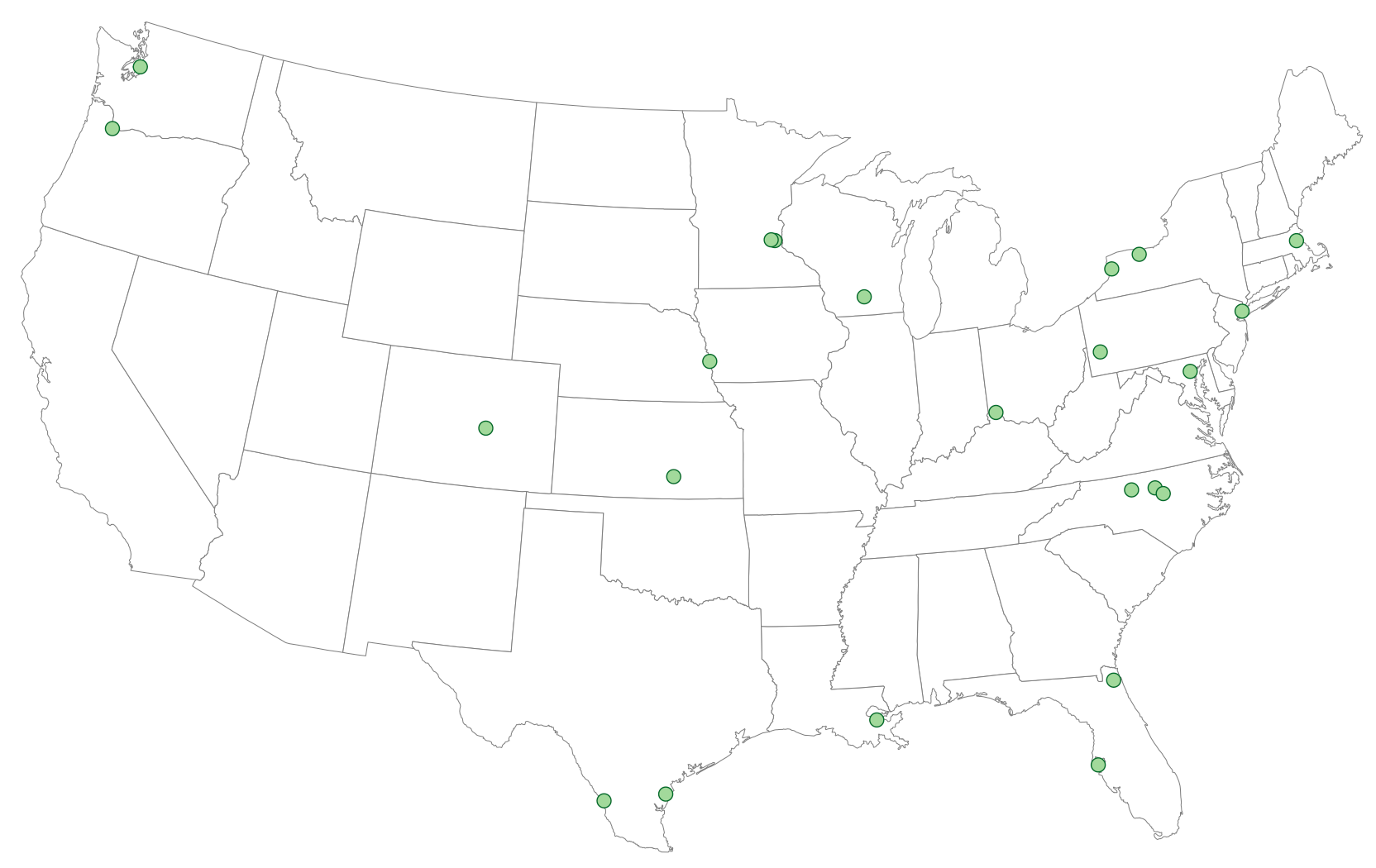Want to share your content on R-bloggers? click here if you have a blog, or here if you don't.

I’ve been seeing an uptick in static US “lower 48″ maps with “meh” projections this year, possibly caused by a flood of new folks resolving to learn R but using pretty old documentation or tutorials. I’ve also been seeing an uptick in folks needing to geocode US city/state to lat/lon. I thought I’d tackle both in a quick post to show how to (simply) use a decent projection for lower 48 US maps and then how to use a very basic package I wrote – localgeo to avoid having to use an external API/service for basic city/state geocoding.

### Albers All The Way

I could just plot an Albers projected map, but it’s more fun to add some data. We’ll start with some setup libraries and then read in some recent earthquake data, then filter it for our map display:

```library(ggplot2) library(dplyr) library(readr) # devtools::install_github("hadley/readr")   # Earthquakes -------------------------------------------------------------   # get quake data ---------------------------------------------------------- quakes <- read_csv("http://earthquake.usgs.gov/earthquakes/feed/v1.0/summary/2.5_month.csv")   # filter all but lower 48 US ---------------------------------------------- quakes %>% filter(latitude>=24.396308, latitude<=49.384358, longitude>=-124.848974, longitude<=-66.885444) -> quakes   # bin by .5 --------------------------------------------------------------- quakes\$Magnitude <- as.numeric(as.character(cut(quakes\$mag, breaks=c(2.5, 3, 3.5, 4, 4.5, 5), labels=c(2.5, 3, 3.5, 4, 4.5), include.lowest=TRUE)))```

Many of my mapping posts use quite a few R geo libraries, but this one just needs `ggplot2`. We extract the US map data, turn it into something `ggplot` can work with, then plot our quakes on the map:

```us <- map_data("state") us <- fortify(us, region="region")   # theme_map --------------------------------------------------------------- devtools::source_gist("33baa3a79c5cfef0f6df")   # plot -------------------------------------------------------------------- gg <- ggplot() gg <- gg + geom_map(data=us, map=us, aes(x=long, y=lat, map_id=region, group=group), fill="#ffffff", color="#7f7f7f", size=0.25) gg <- gg + geom_point(data=quakes, aes(x=longitude, y=latitude, size=Magnitude), color="#cb181d", alpha=1/3) gg <- gg + coord_map("albers", lat0=39, lat1=45) gg <- gg + theme_map() gg <- gg + theme(legend.position="right") gg```

2.5+ mag quakes in Lower US 48 in past 30 days### Local Geocoding

There are many APIs with corresponding R packages/functions to perform geocoding (one really spiffy recent one is geocodeHERE). While Nokia’s service is less restrictive than Google’s, most of these sites are going to have some kind of restriction on the number of calls per second/minute/day. You could always install the Data Science Toolkit locally (note: it was down as of the original posting of this blog) and perform the geocoding locally, but it does take some effort (and space/memory) to setup and get going.

If you have relatively clean data and only need city/state resolution, you can use a package I made – localgeo as an alternative. I took a US Gov census shapefile and extracted city, state, lat, lon into a data frame and put a lightweight function shim over it (it’s doing nothing more than `dplyr::left_join`). It won’t handle nuances like “St. Paul, MN” == “Saint Paul, MN” and, for now, it requires you to do the city/state splitting, but I’ll be tweaking it over the year to be a bit more forgiving.

We can give this a go and map the greenest cities in the US in 2014 as crowned by, er, Nerd Wallet. I went for “small data file with city/state in it”, so if you know of a better source I’ll gladly use it instead. Nerd Wallet used DataWrapper, so getting the actual data was easy and here’s a small example of how to get the file, perform the local geocoding and use an Albers projection for plotting the points. The code below assumes you’re still in the R session that used some of the `library` calls earlier in the post.

```library(httr) library(localgeo) # devtools::install_github("hrbrmstr/localgeo") library(tidyr)   # greenest cities --------------------------------------------------------- # via: http://www.nerdwallet.com/blog/cities/greenest-cities-america/   url <- "https://gist.githubusercontent.com/hrbrmstr/1078fb798e3ab17556d2/raw/53a9af8c4e0e3137a0a8d4d6332f7a6073d93fb5/greenest.csv" greenest <- read.table(text=content(GET(url), as="text"), sep=",", header=TRUE, stringsAsFactors=FALSE)   greenest %>% separate(City, c("city", "state"), sep=", ") %>% filter(!state %in% c("AK", "HI")) -> greenest   greenest_geo <- geocode(greenest\$city, greenest\$state)   gg <- ggplot() gg <- gg + geom_map(data=us, map=us, aes(x=long, y=lat, map_id=region, group=group), fill="#ffffff", color="#7f7f7f", size=0.25) gg <- gg + geom_point(data=greenest_geo, aes(x=lon, y=lat), shape=21, color="#006d2c", fill="#a1d99b", size=4) gg <- gg + coord_map("albers", lat0=39, lat1=45) gg <- gg + labs(title="Greenest Cities") gg <- gg + theme_map() gg <- gg + theme(legend.position="right") gg```

Nerd Wallets’s Greenest US (Lower 48) Cities 2014Let me reinforce that the `localgeo` package will most assuredly fail to geocode some city/state combinations. I’m looking for a more comprehensive shapefile to ensure I have the complete list of cities and I’ll be adding some code to help make the lookups more forgiving. It may at least help when you bump into an API limit and need to crank out something in a hurry.